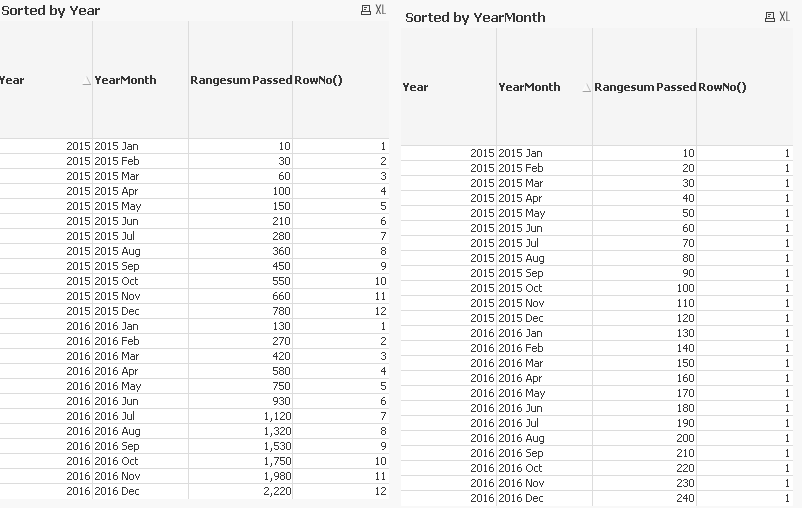# QlikView App Development

Discussion Board for collaboration related to QlikView App Development.

HighlightedLuminary

## Aggr(Rangesum(above(sum

Hi,

I´ve a nice aggr(rangesum(above example attached. What I don´t get yet is the following: In my example file you find a column in the left straight table that includes the column "Rangesum Passed per Year". The result is already what I want. But I do not want to see only the totals. And the totals also does not make sense in the first month of the year. I want the aggregation for each of the months and then stop when the year switches and start over with the next year. Is this possible?

6 Replies
HighlightedLuminary

## Re: Aggr(Rangesum(above(sum

I detected another issue. It looks like as soon as the column "Rangesum Passed per Year" is activated it changes my values in the other columns like "Rangesum Passed", "Rangesum Total" and "Rangesum % Calculated". This is strange. Why is it affecting it?

HighlightedMVP & Luminary

## Re: Aggr(Rangesum(above(sum

No idea. After disabling and re-enabling the fourth expression (also called Rangesum Passed just like the first expression) it all looks fine to me. The running total resets on year borders.

You could add a nodistinct in the aggr of the fourth expression so you get the year total at every row.

talk is cheap, supply exceeds demand
HighlightedLuminary

## Re: Aggr(Rangesum(above(sum

If I enable the "Rangesum Passed per Year" it changes the column "Rangesum Passed" and "Rangesum Total". If it is enabled the values get incorrect if it is disabled the other two expressions are correct again.

Is it

=Num(nodistinct(Aggr(Rangesum(Above(sum({\$<Result = {'Passed'}>}Quantity),0,1)),Year)),\$(vInteger))

then ? I do not want the same total in every row. I want it to be aggregated step by step and then stop at the end ot the last preriod of the year. Then it should start over with the next year.Luminary

## Re: Aggr(Rangesum(above(sum

Strange, this should work OK.

You can work with TOTAL qualifier and make the Above function go as many steps up as needed.

`Num(Rangesum(Above(TOTAL sum({\$<Result = {'Passed'}>}Quantity),0,If(Mod(RowNo(TOTAL), 12)=0, 12, Mod(RowNo(TOTAL), 12)))),\$(vInteger))`

This will work only for full years, but I guess it could be adjusted with GetPossibleCount(YearMonth).

HighlightedLuminary

## Re: Aggr(Rangesum(above(sum

Plus, I noticed that the result of the first expression is affected by sorting. If you sort by Year, it works fine. If you sort by YearMonth,  it does not work. I guess this affects how Above() handles dimensions and whether or not the function is in fact "able" to see on the row above it without TOTAL qualifier.

My way of debugging expressions like this is adding a dummy expression containing only RowNo() to see how dimensions and sort order affects the results.HighlightedMVP & Luminary

## Re: Aggr(Rangesum(above(sum

Ok, it's the interactive sorting that causing the problem. For the rangesum(above( ... )) construct to work correctly the data must be sorted by Year first and YearMonth secondly. Otherwise every YearMonth will be a border where the running total gets reset. So you need to fix the sort order on the Sort tab and disable the Allow Interactive Sort option.

talk is cheap, supply exceeds demand